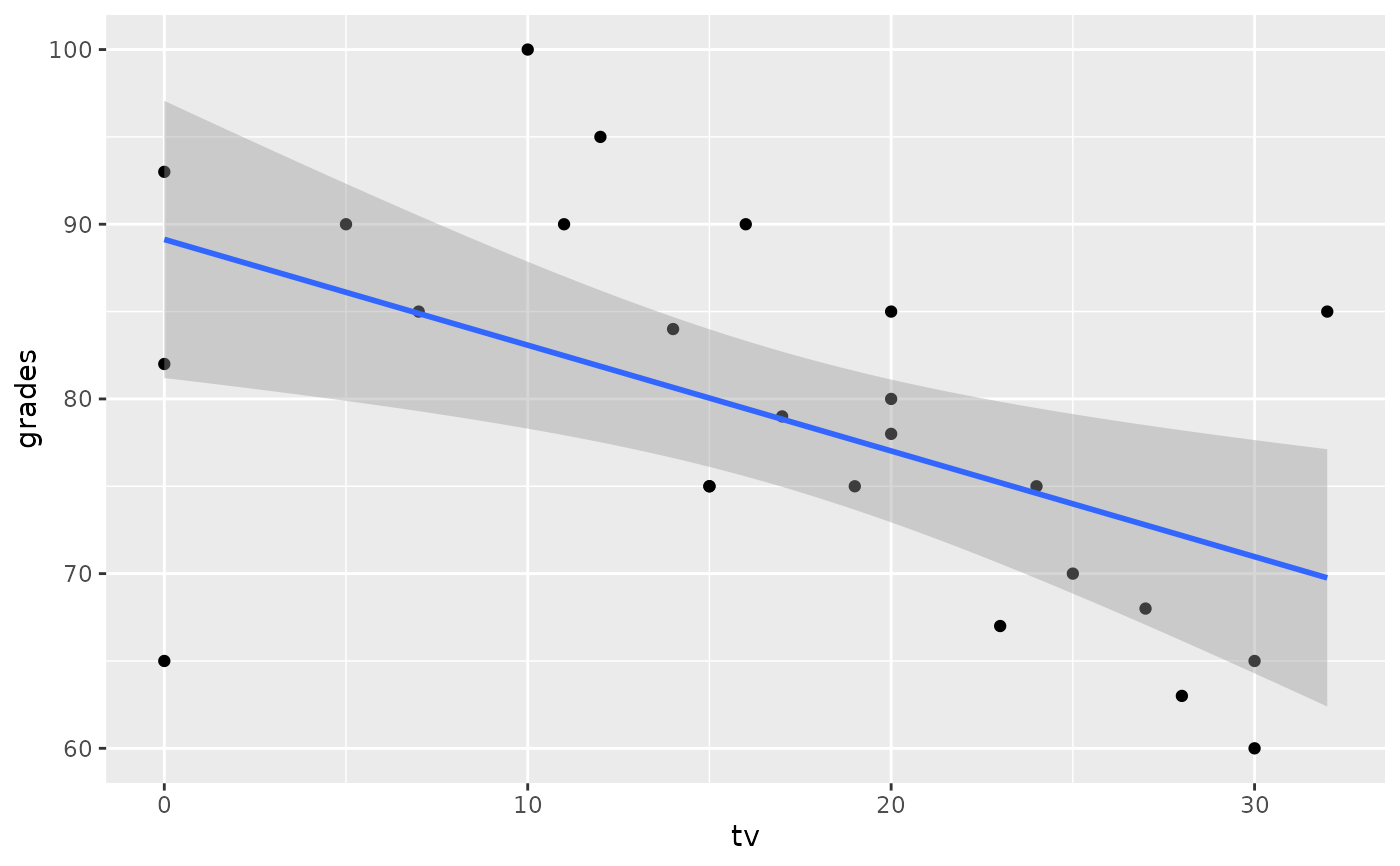This is a simulated data set to be used to estimate the relationship between number of hours per week students watch TV and the grade they got in a statistics class.

## Usage

gradestv

## Format

A data frame with 25 observations on the following 2 variables.

tv

Number of hours per week students watch TV.

Grades students got in a statistics class (out of 100).

Simulated data

## Details

There are a few potential outliers in this data set. When analyzing the data one should consider how (if at all) these outliers may affect the estimates of correlation coefficient and regression parameters.

## Examples


library(ggplot2)

#> geom_smooth() using formula = 'y ~ x'